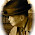## Monday, December 10, 2012

### Leo Tolstoy and Mathematics

Leo Tolstoy. By Scan by User: Gabor [Public domain], via Wikimedia Commons.

These days I am reading Leo Tolstoy's autobiographical novels Childhood, Boyhood and Youth by Japanese translations in Shinchō World Literature Volume 16 (1972). In the first half of Youth, there is an episode that the hero, "I", passes the entrance examination to the mathematics department of a university.

The examination is made in the following manner: Each applicant takes a piece from the problem cards held by a professor and answers to the problem chosen, in front of the professor. In the mathematics examination, there were two professors. The hero was called at the same time as another applicant, and they secretly exchanged the cards they chose. The card the hero initially took was of the problem about "combinatorics," which he thought difficult. However, the question he got by exchanging the cards was about "Newton's binomial theorem," which he had tried to solve just before the examination. Thus, the hero was able to answer it perfectly.

Reading this, you may wonder if Tolstoy studied in the mathematics department. In fact, Tolstoy began studying law and oriental languages at Kazan University and left university in the middle of his studies (Ref. 1). Therefore, the story of the entrance to the mathematics department is fictional.

I learned "combinatorics" and "the binomial theorem" in senior high school. However, the latter was not Newton's but the basic one. The basic "binomial theorem" describes a formula for the algebraic expansion of nth power of a binomial x + y, where n is a positive integer. Isaac Newton generalized the formula to allow real exponents, and the formula can be generalized further, to complex exponents (Ref. 2).

It was probably at university that I learned about generalized binomial theorem. Therefore, Newton's binomial theorem seems to be too difficult for the entrance examination of university. It is also strange that the hero thought Newton's binomial theorem easier than combinatorics. This is because we learn combinatorics as preparation for studying the basic binomial theorem. Thus, I guess that problems of mathematics were also invented by Tolstoy on the basis of his superficial knowledge of these terms of mathematics. What do you think?

References
1. "Leo Tolstoy," Wikipedia: The Free Encyclopedia (December 8, 2012 at 19:10).
2. "Binomial theorem," Wikipedia: The Free Encyclopedia (November 25, 2012 at 04:39).

1.2.